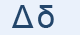Greek Letter Delta (Δέλτα - Δ)"Delta" was supposed to be a door, in Semitic "Daleth". It became tilted and one side rounded as the latin "D". The mouth of a river and maybe other triangular things are known as "deltas".

"Δ" Letter in mathematics and in science

Δ represents:

• a finite difference
• a difference operator
• the Laplace operator
• the angle that subtends the arc of a circular curve in surveying

δ represents:

• a variation in the calculus of variations
• the Kronecker delta function
• the Dirac delta function
• sensitivity to price in mathematical finance

Greek words with "δ"

δώρο, δωμάτιο